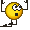# Math Challenge - April 2021

• Challenge
statement one:
Show that all Pythagorean triples ##x^2 + y^2 = z^2## can be found by
##(x, y , z) = (u^2 - v^2, 2uv, u^2 + v^2)## with ##u, v \in \mathbb{N} , u > v##

Sorry again, I am lost. I was able to find a way by which any primitive Pythagorean triple can be expressed as ##(u^2 - v^2, 2uv, u^2 + v^2)## with ##u, v \in \mathbb{N}## and that part of the proof was accepted, but I am unable to understand how and why all non-primitive triples should be expressible the same way. I take the same example of non-primitive Pythagorean triple as in an earlier reply, ##(9, 12, 15)##. Since 12 is the only even number in this triple, only it can be expressed as ##2uv## for some natural numbers ##u,v##. That leaves 9 to be expressed as ##u^2 - v^2##. The possible solutions for ##12 = 2uv## are ##(u=1, v=6)##, ##(u=2, v=3)##, ##(u=3, v=2)##, ##(u=6, v=1)##. In none of these solutions do I get ##u^2 - v^2 = 9##. In other words, ##(9, 12, 15)## appears to be a counterexample for ##(x, y , z) = (u^2 - v^2, 2uv, u^2 + v^2)##. What am I missing?

Mentor
Sorry again, I am lost. I was able to find a way by which any primitive Pythagorean triple can be expressed as ##(u^2 - v^2, 2uv, u^2 + v^2)## with ##u, v \in \mathbb{N}## and that part of the proof was accepted, but I am unable to understand how and why all non-primitive triples should be expressible the same way. I take the same example of non-primitive Pythagorean triple as in an earlier reply, ##(9, 12, 15)##. Since 12 is the only even number in this triple, only it can be expressed as ##2uv## for some natural numbers ##u,v##. That leaves 9 to be expressed as ##u^2 - v^2##. The possible solutions for ##12 = 2uv## are ##(u=1, v=6)##, ##(u=2, v=3)##, ##(u=3, v=2)##, ##(u=6, v=1)##. In none of these solutions do I get ##u^2 - v^2 = 9##. In other words, ##(9, 12, 15)## appears to be a counterexample for ##(x, y , z) = (u^2 - v^2, 2uv, u^2 + v^2)##. What am I missing?
You are right. I made a mistake (sloppy translation). It should have been ... of the form ##d\cdot (u^2-v^2,2uv,u^2+v^2)## ...

I apologize for that negligence, @Not anonymous.

I apologize for that negligence, @Not anonymous.
No problem (problem ≠ math problem in this context). Thanks to you as always for promptly reviewing my answers and patiently explaining what I may have missed or got wrong.•fresh_42